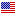1st Edition

# Techniques for Designing and Analyzing Algorithms

By Douglas Robert Stinson Copyright 2021
ISBN 9780367228897
444 Pages 66 Color Illustrations
Published August 6, 2021 by Chapman & HallFree Shipping (6-12 Business Days)
shipping options

Original Price \$110.00
Sale Price USD \$88.00
ISBN 9780429277412
444 Pages 66 Color Illustrations
Published August 5, 2021 by Chapman & Hall

Also available as eBook on:

Techniques for Designing and Analyzing Algorithms

Design and analysis of algorithms can be a difficult subject for students due to its sometimes-abstract nature and its use of a wide variety of mathematical tools. Here the author, an experienced and successful textbook writer, makes the subject as straightforward as possible in an up-to-date textbook incorporating various new developments appropriate for an introductory course.

This text presents the main techniques of algorithm design, namely, divide-and-conquer algorithms, greedy algorithms, dynamic programming algorithms, and backtracking. Graph algorithms are studied in detail, and a careful treatment of the theory of NP-completeness is presented.

In addition, the text includes useful introductory material on mathematical background including order notation, algorithm analysis and reductions, and basic data structures. This will serve as a useful review and reference for students who have covered this material in a previous course.

Features

• The first three chapters provide a mathematical review, basic algorithm analysis, and data structures
• Detailed pseudocode descriptions of the algorithms along with illustrative algorithms are included
• Proofs of correctness of algorithms are included when appropriate
• The book presents a suitable amount of mathematical rigor

After reading and understanding the material in this book, students will be able to apply the basic design principles to various real-world problems that they may encounter in their future professional careers.

1. Introduction and Mathematical Background. 1.1. Algorithms and Programs. 1.2. Definitions and Terminology. 1.3. Order Notation. 1.4. Mathematical Formulae. 1.5. Probability Theory and Random Variables. 1.6. Notes and References. Exercises. 2. Algorithm Analysis and Reductions. 2.1. Loop Analysis Techniques. 2.2. Algorithms for the 3SUM Problem. 2.3. Reductions. 2.4. Exact Analysis. 2.5. Notes and References. Exercises. 3. Data Structures. 3.1. Abstract Data Types and Data Structures. 3.2. Arrays, Linked Lists and Sets. 3.3. Stacks and Queues. 3.4. Priority Queues and Heaps. 3.6. Hash Tables. 3.7. Notes and References. Exercises. 4. Divide-and-Conquer Algorithms. 4.1. Recurrence Relations. 4.2. The Master Theorem. 4.3. Divide-and-Conquer Design Strategy. 4.4. Divide-and-Conquer Recurrence Relations. 4.5. Binary Search. 4.6. Non-dominated Points. 4.7. Stock Profits. 4.8. Closest Pair. 4.9. Multiprecision Multiplication. 4.10. Modular Exponentiation. 4.11. Matrix Multiplication. 4.12. QuickSort. 4.13. Selection and Median. 4.14. Notes and References. Exercises. 5. Greedy Algorithms. 5.1. Optimization Problems. 5.2. Greedy Design Strategy. 5.3. Interval Selection. 5.4. Interval Coloring. 5.5. Wireless Access Points. 5.6. A House Construction Problem. 5.7. Knapsack. 5.8. Coin Changing. 5.9. Multiprocessor Scheduling. 5.10. Stable Matching. 5.11. Notes and References. Exercises. 6 Dynamic Programming Algorithms. 6.1. Fibonacci Numbers. 6.2. Design Strategy. 6.3. Treasure Hunt. 6.4. 0-1 Knapsack. 6.5. Rod Cutting. 6.6. Coin Changing. 6.7. Longest Common Subsequence. 6.8. Minimum Length Triangulation. 6.9. Memoization. 6.10. Notes and References. Exercises. 7. Graph Algorithms. 7.1. Graphs. 7.2. Breadth-first Search. 7.3. Directed Graphs. 7.4. Depth-first Search. 7.5. Strongly Connected Components. 7.6. Eulerian Circuits. 7.7. Minimum Spanning Trees. 7.8. Single Source Shortest Paths. 7.9. All-Pairs Shortest Paths. 7.10. Notes and References. Exercises. 8. Backtracking Algorithms. 8.1. Introduction. 8.2. Generating all Cliques. 8.3. Sudoku. 8.4. Pruning and Bounding Functions. 8.5. 0-1 Knapsack Problem. 8.6. Traveling Salesperson Problem. 8.7. Branch-and-bound. 8.8. Notes and References. Exercises. 9. Intractability and Undecidability. 9.1. Decision Problems and the Complexity Class P. 9.2. Polynomial-time Turing Reductions. 9.3. The Complexity Class NP. 9.4. Polynomial Transformations. 9.5. NP-completeness. 9.6. Proving Problems NP-complete. 9.7. NP-hard Problems. 9.8. Approximation Algorithms. 9.9. Undecidability. 9.10. The Complexity Class EXPTIME. 9.11. Notes and References. Exercises. Bibliography. Index.

### Biography

Douglas R. Stinson obtained his PhD in Combinatorics and Optimization from the University of Waterloo in 1981. He held academic positions at the University of Manitoba and the University of Nebraska-Lincoln before returning to Waterloo in 1998. In 2019, Dr. Stinson retired from the David R. Cheriton School of Computer Science at the University of Waterloo and he now holds the title Professor Emeritus. His research interests include cryptography and computer security, combinatorics and coding theory, and applications of discrete mathematics in computer science. He was elected as a Fellow of the Royal Society of Canada in 2011. He has published almost 400 papers and several books, including Cryptography: Theory and Practice, Fourth Edition, also published by CRC Press.

We offer free standard shipping on every order across the globe.